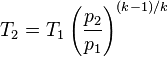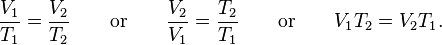# Thermodynamics help please. Compression, heat, cool, decompression

• InquisitiveOne

#### InquisitiveOne

Hello everyone. New guy, first post.

I typically frequent gasoline engine performance forums, but my inquisitive nature has led me beyond the scope of general knowledge available within that format. I appreciate any help in figuring out the following.

If 100 cubic feet of air at sea level (about 15psia) is 70°F and is compressed to 60psig (75psia), what is the resultant volume and temperature? (assuming no outside losses or gains)

If the temperature of the compressed air is reduced to the original temp of 70°, but the pressure of 60psig is maintained, what is the new volume?

Lastly, if the air, compressed to 60psig @ 70°F, is decompressed to 15psig (30psia), what is it's resultant temperature?

Recap:
Air at 70°F - Compressed 60psi over ambient- cooled - decompressed to 15psi over ambient... What's the new temp?

A ton of thanks to any input!

Last edited:

Is this the correct equation to use in finding temperature increase with compression?T1 = 70°F = 294.261kelvin
P1 = 14.7psi or 1 bar
P2 = 73.5 psi or 5 bar absolute pressure

In reading about the value for k, I've found that most use 1.4, which is for dry air at 20°c. I've noted that the value for just air (not dry air) is 1.67 at 20°c. Is there one value I should use over the other? Or a certain relative humidity when I should use one over the other?

It's been quite some time since I've done this kind of stuff, any help/advice is much appreciated.

1.67 is for a monatomic ideal gas, while 1.4 is for a diatomic gas (and since nitrogen and oxygen are both diatomic, 1.4 is pretty much perfect for air). I'm not sure why anyone would use 1.67 for air - any deviations from 1.4 for air (including humidity effects) would tend to make it lower, not higher. My guess is that the 1.67 value is simply incorrect - maybe someone was looking at the value for a monatomic gas (helium, argon, etc) instead.

As for that formula? Yes, it should be correct. You can also use PVγ = constant (γ is the same parameter as your k - I'm just more used to seeing it as γ rather than k).

My guess is that the 1.67 value is simply incorrect - maybe someone was looking at the value for a monatomic gas (helium, argon, etc) instead.

Thank you for your time. I went back to the reference I used to find the Heat Capacity Ratio, and you are completely correct. I mistakenly read Ar as Air.When you say "no outside losses or gains" - that means "adiabatic". I agree, for air, 1.4 is the number to use for k, more commonly called $\gamma$

Maybe it's appropriate for this to be in the homework area. I'm drawing on memory and can't seem to work the equation out in any way that makes sense. A little embarrassing to have to ask, but would one of you guys walk me through it? Please.

Did it again. Came up with 466.27 kelvin (379.616F)

Does that seem right?

Did it again. Came up with 466.27 kelvin (379.616F)

Does that seem right?T1 = 70°F = 294.261kelvin
P1 = 14.7psi or 1 bar
P2 = 73.5 psi or 5 bar absolute pressure

I solved for the exponent first, 1.4 -1 = 0.4 / 1.4 = 0.286
Then solved the pressure ratio, 73.5 / 14.7 = 5
Pressure ratio of 5 to the power of 0.286 = 1.585
1.585 x 294.26 kelvin = 466 kelvin

Is that correct?

Thanks for your help by the way.T1 = 70°F = 294.261kelvin
P1 = 14.7psi or 1 bar
P2 = 73.5 psi or 5 bar absolute pressure

I solved for the exponent first, 1.4 -1 = 0.4 / 1.4 = 0.286
Then solved the pressure ratio, 73.5 / 14.7 = 5
Pressure ratio of 5 to the power of 0.286 = 1.585
1.585 x 294.26 kelvin = 466 kelvin

Is that correct?

Thanks for your help by the way.

That looks good.

I agree with rap - that looks good. You can use the same technique for the second part of your question as well. That temperature is low enough that dry air should pretty much act ideally (so you don't need to worry about deviations from ideal gas behavior) too, so unless you've got an extremely high humidity, that should be a pretty accurate result.

That looks good.
Rap, I can't thank you enough.

Reading through Wikipedia and taking notes like a man on a mission, I came upon this equation which I believe converts the numbers I have so far into a volumetric compression ratio, or pressure ratio to compression ratio.

CR = T1 / T2 x PR

I checked it against a chart on the page and seems to work out.

If:
T1 = 294
T2 = 466
PR = 5

294 / 466 x 5 = 3.15:1 Compression Ratio

So if the original volume was 100 cubic feet the new compressed volume is 31.75 cubic feet.

Is this correct?

If the above checks out, can I apply Charles's Law to find the volume once the heat of compression is removed?If,
V1 = 31.75 cubic feet
T1 = 466
T2 = 294

Then, 31.75 / 466 x 294 = 20 cubic feet

So, the new compression ratio, assuming a constant pressure ratio of 5 is now 5:1?

Interesting. If all the calculations are correct... very interesting.

I agree with rap - that looks good. You can use the same technique for the second part of your question as well. That temperature is low enough that dry air should pretty much act ideally (so you don't need to worry about deviations from ideal gas behavior) too, so unless you've got an extremely high humidity, that should be a pretty accurate result.

I was writing when you posted and missed your reply earlier. Thank you for the verification and input.

So, I can use the same equation to find the temperature upon decompression? Let's try it out!k = 1.4 so the exponent is 0.286
P2 = 29.4 absolute (14.7 psig)
P1 = 73.5
T1 = 294

So, 29.4 / 73.5 to the power of 0.286 = 0.7695
0.7695 x 294 = 226 kelvin = -53°F

If all the above is sound and correct, that's pretty awesome!

Thank you cjl!

Lets call $R_T=T_2/T_1$ the temperature ratio, $R_P=P_2/P_1$ the pressure ratio, $R_V=V_2/V_1$ the volume ratio. Then the ideal gas law says: $$R_P R_V=R_T$$ and adiabatic says $$R_P R_V^k$$ and that will give you everything you need, like $R_T =R_P^{(k-1)/k}$ that you used before. The equation you need is from the first one: $$R_V=R_T/R_P$$We have $R_P=5$ and $R_T=1.58$, so $R_V=1.58/5=1/3.15$ and so $V_2=V_1/3.15=31.7$, so yes, you got it right.

It looks like what you are calling the compression ratio CR is $1/R_V$, but I don't know why.

Lets call $R_T=T_2/T_1$ the temperature ratio, $R_P=P_2/P_1$ the pressure ratio, $R_V=V_2/V_1$ the volume ratio. Then the ideal gas law says: $$R_P R_V=R_T$$ and adiabatic says $$R_P R_V^k$$ and that will give you everything you need, like $R_T =R_P^{(k-1)/k}$ that you used before. The equation you need is from the first one: $$R_V=R_T/R_P$$We have $R_P=5$ and $R_T=1.58$, so $R_V=1.58/5=1/3.15$ and so $V_2=V_1/3.15=31.7$, so yes, you got it right.

Woo Hoo! (doing a little victory dance)It looks like what you are calling the compression ratio CR is $1/R_V$, but I don't know why.

In automotive engine vernacular, what you call volume ratio, is referred to as the compression ratio and is usually expressed as 3.15:1 (in this case). Until now, that's the only term I knew to use.

Last edited:
Been trying to figure this out all day and the light bulb just came on. Just going to jot it down here for my future reference...

Figuring out pressure and pressure ratio with a known compression ratio/volume ratio.

Simple really. It's the ratio to the power of 1.4

10:1
10 to the power of 1.4 = 25.12 PR

To find pressure:
25.12 x P1 = P2

To find Temperature:
25.12 to the power of 0.286 x T1 = T2

Guys, really... Thank you for all the help.